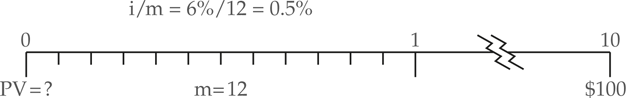# Present Value with Non-Annual Compounding

When the compounding period is not annual we must be sure to use a periodic interest rate that reflects the length of the period. The periodic rate is simply i/m, where m is the number of compounding periods in a year. For example, if the nominal rate is 10% (i=10%) and the compounding period is semi-annual (m=2), then the periodic rate is 10%/2 or 5%.

Formula

The formula for present value with non-annual compounding is analogous to the formula for the future value with non-annual compounding:

$P{{V}_{0}}=F{{V}_{n}}\times \frac{1}{{{\left( 1{{+}^{i}}{{/}_{m}} \right)}^{n\times m}}}$

Example 1 Solving for the Present Value with Non-Annual Compounding\begin{align} & FV=\100\\&i=0.06\\&n=10years\\&n*m=1\text{20months}\\&i/m=0.06/12=0.005/month\\\end{align}
\begin{align} & P{{V}_{o}}=F{{V}_{n}}*\frac{1}{{{\left( 1+{}^{i}/{}_{m} \right)}^{n*m}}} \\ & P{{V}_{o}}=\100*\frac{1}{{{1.005}^{120}}}=\54.96\\\end{align}